## Graduate Students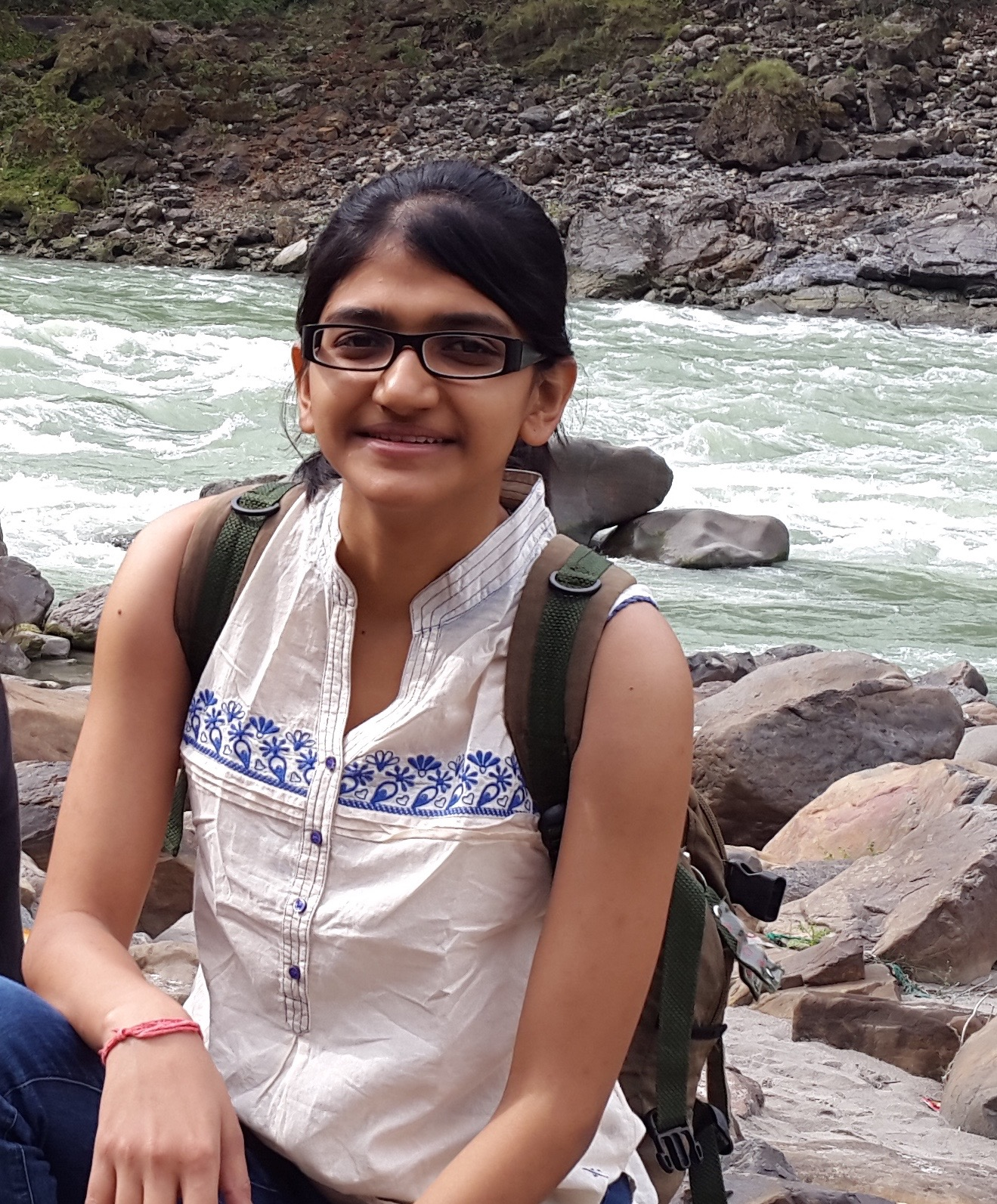Sumegha Garg
Complexity Theory
Information Theory
Algorithmic Fairness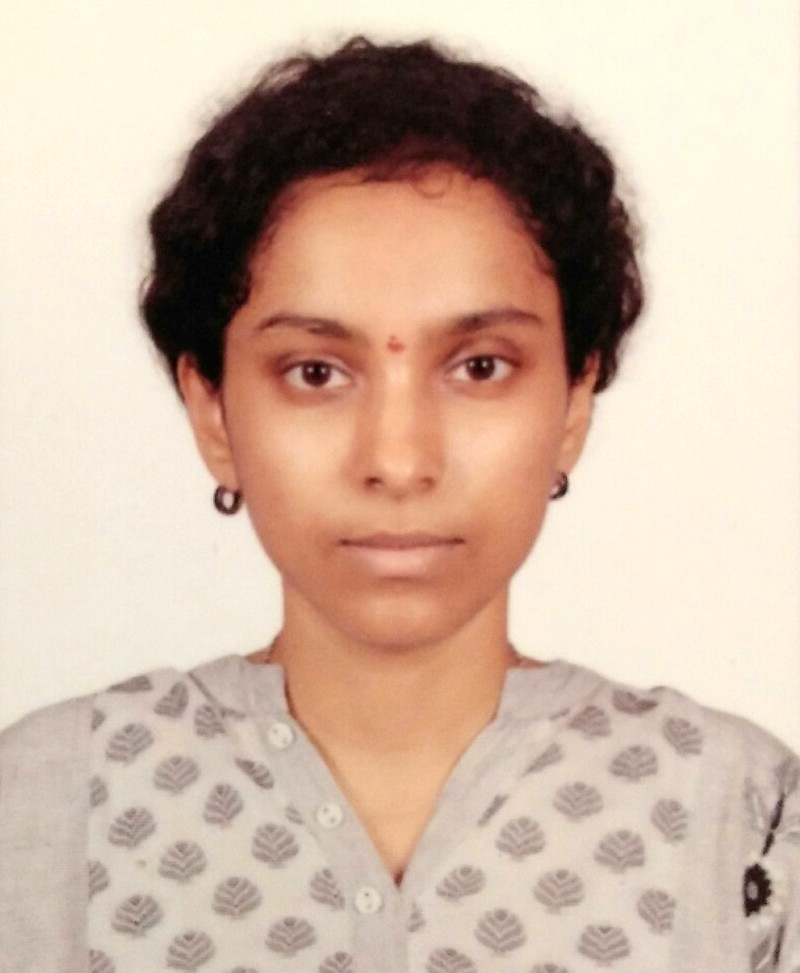Uma Girish
Complexity Theory
Combinatorics
PseudorandomnessWei Hu
Theoretical Machine Learning
Optimization
Algorithms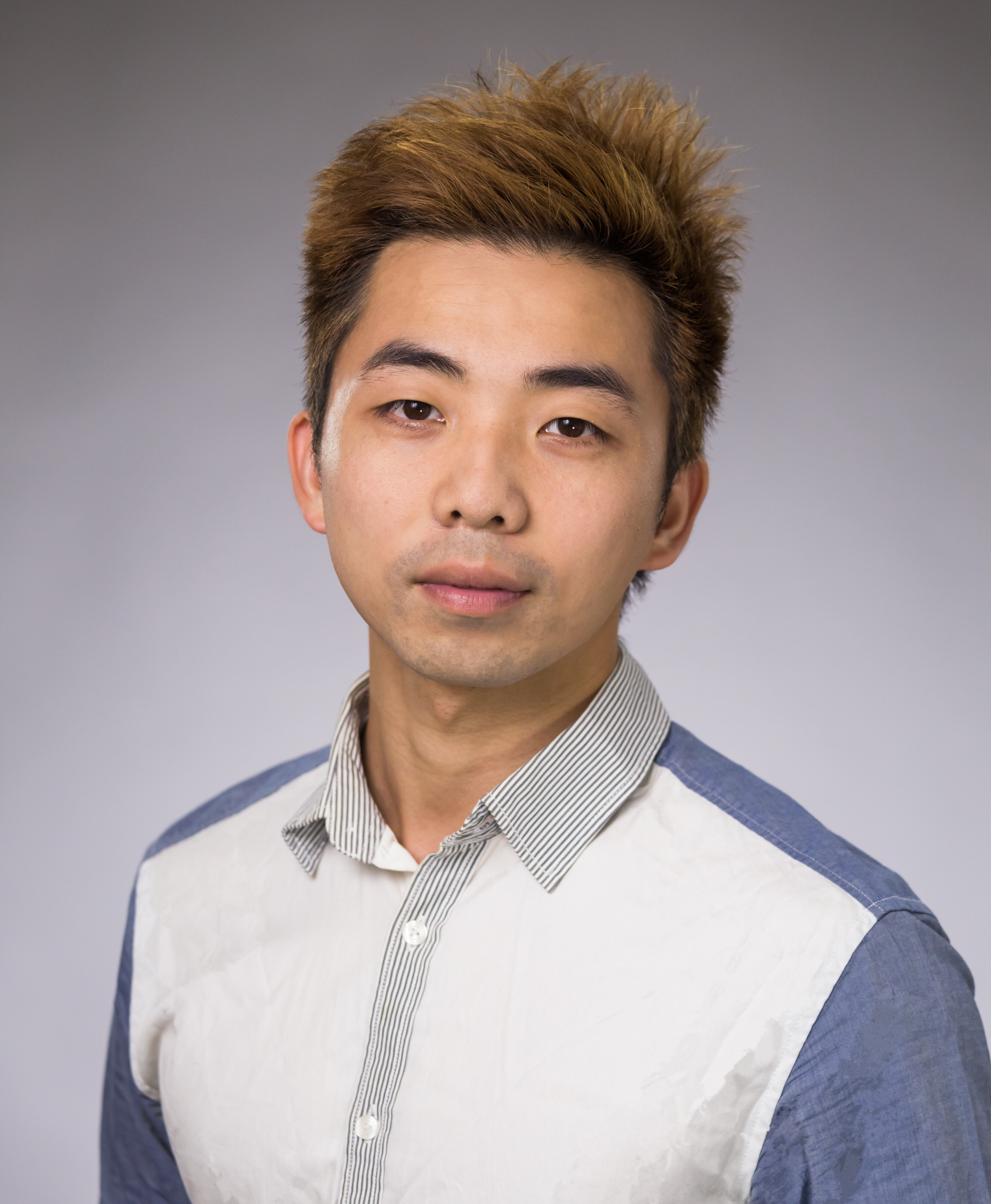Sixue Liu
Algorithms and Data Structures
Combinatorics
Computational ComplexityFermi Ma
Program Obfuscation
Public-Key Cryptography
AlgorithmsEdgar Minasyan
Theoretical Machine Learning
Optimization
AlgorithmsClay Thomas
Algorithmic Game Theory
Complexity Theory
Coding Theory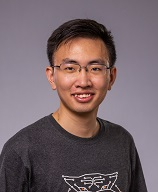Dingli Yu
Algorithmic Game Theory
Theoretical Mechining Learning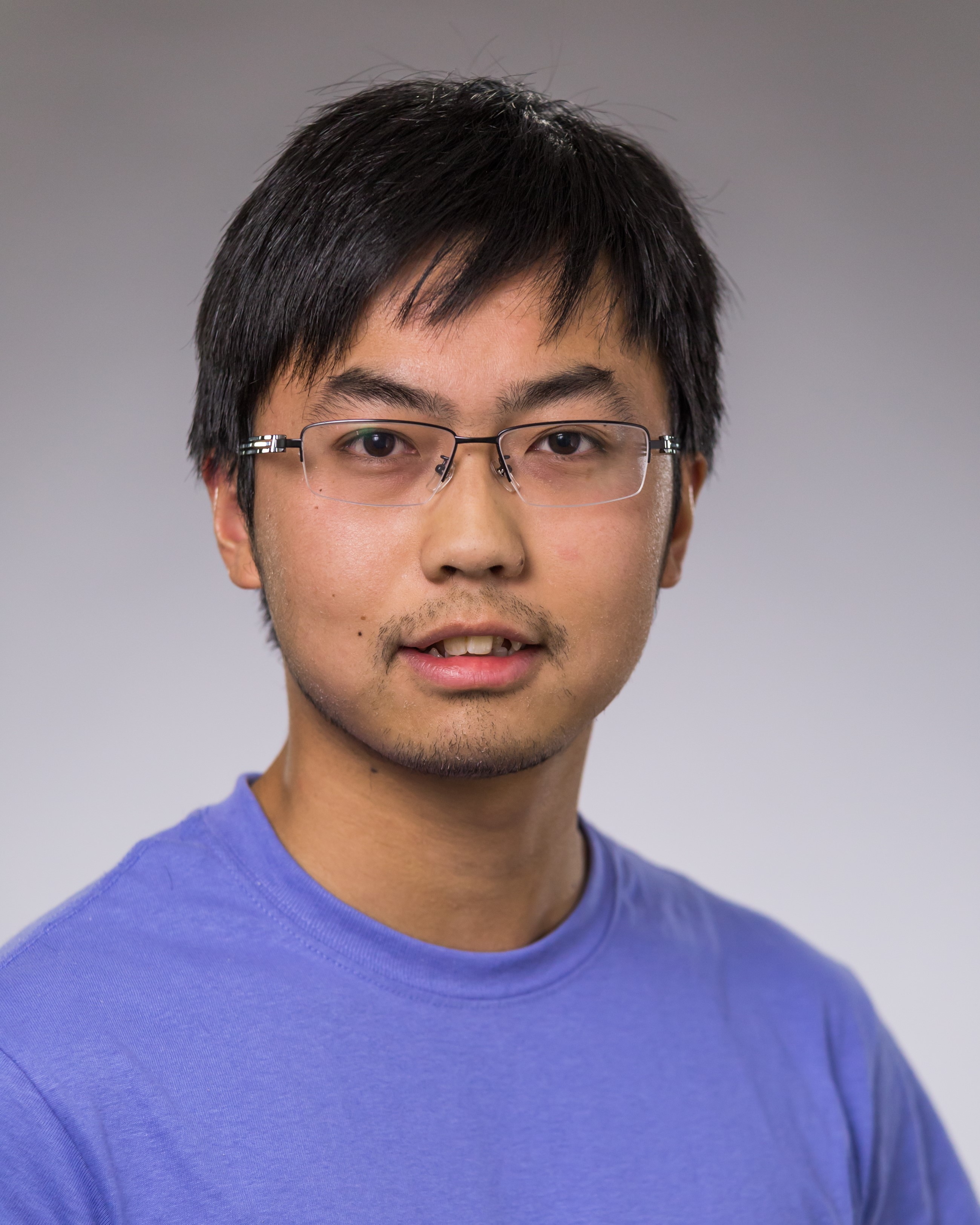Wei Zhan
Complexity Theory
Computational Geometry
Combinatorics